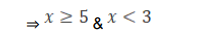# Find the domain and the range of each of the following real

Question:

Find the domain and the range of each of the following real

function: $f(x)=\sqrt{\frac{x-5}{3-x}}$

Solution:

$f(x)=\sqrt{\frac{x-5}{3-x}}$

Need to find: Where the functions are defined

The condition for the function to be defined,

$3-x>0$

$\Rightarrow^{x<3}$

So, the domain of the function is the set of all the real numbers lesser than 3 .

The domain of the function, $\mathrm{D} \mathrm{f}(x)=(-\infty, 3)$.

The condition for the range of the function to be defined,

$x-5 \geq 0 \&^{3-x}>0$Both the conditions can’t be satisfied simultaneously. That means there is no range for the function f(x).